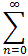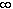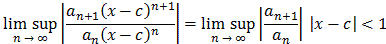#Interactive Real Analysis

Next | Previous | Glossary | Map

## 8.3. Series and Power Series

### Theorem 8.3.7: Power Series

Every power seriesan (x - c)n = a0 + a1(x-c) + a0(x-c)2 + ... centered at c has the following properties:
1. The power series converges at its center, i.e. for x = c
2. There exists an r such that the series converges absolutely and uniformly for all |x - c|p, where p < r, and diverges for all |x - c| > r. The number r is called the radius of convergence for the power series and is given by:
r = lim inf | an / an+1|
Note that it is possible for the radius of convergence to be zero (i.e. the power series converges only for x = c) or to be(i.e. the series converges for all x).

This theorem is sometimes called Abel's theorem on Power Series.

Clearly the power series converges for x = c since then all terms except the first reduce to zero. For the second statement, we will simply apply the Ratio test for series:

The seriesan (x - c)n converges absolutely if:But then, taking the reciprocal:which proves that the series converges absolutely for |x - c| < r. The fact that it converges uniformly on any closed disk centered at c with radius p < r follows from the Weierstrass Convergence theorem.Next | Previous | Glossary | Map